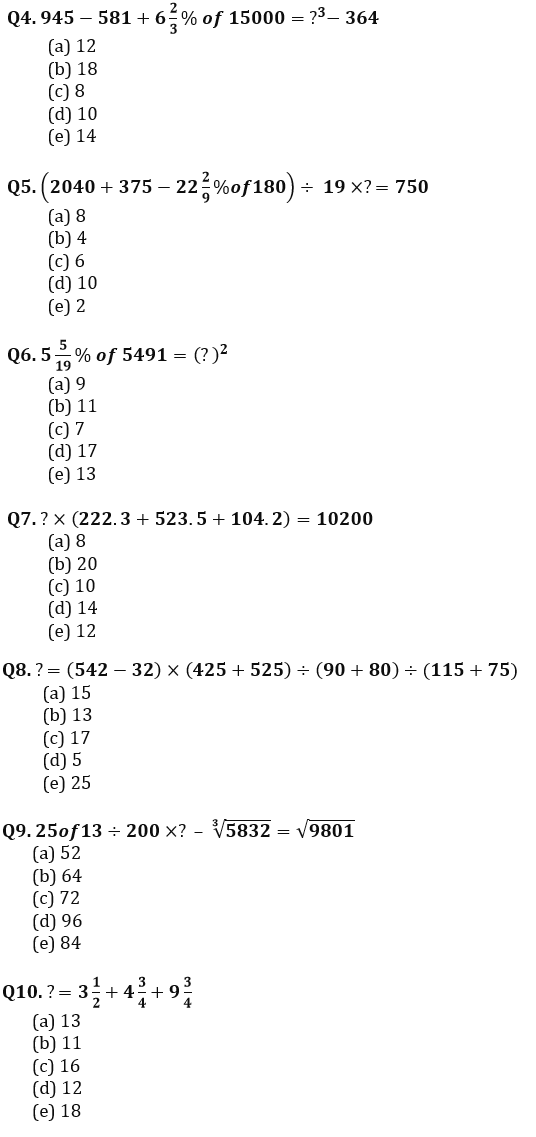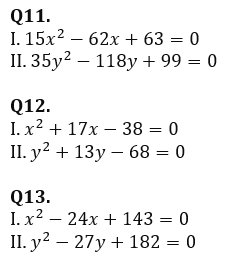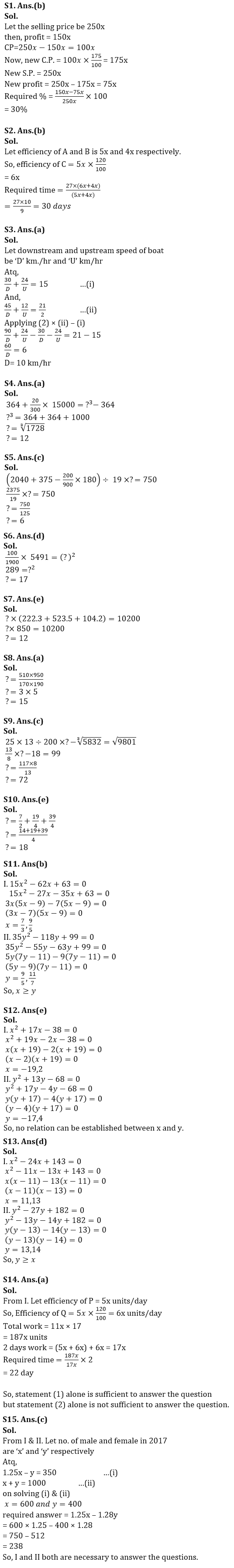Latest Banking jobs   »

# Quantitative Aptitude Quiz For FCI Phase I 2022- 25th December

Q1. When a person sold an article, his profit percent is 60% of the selling price. If the cost price is increased by 75% and the selling price remains the same, then find decrement in the profit is what percent of the selling price of the article?
(a) 25%
(b) 30%
(c) 40%
(d) 27.5%
(e) None of these

Q2. Ratio of efficiency of A and B is 5 : 4, if efficiency of C is 20% more than A. And if B and C together can complete the work in 27 days, find time taken by A and B together to complete the same work?
(a) 45 days
(b) 30 days
(c) 35 days
(d) 40 days
(e) none of these

Q3. A boat can cover 30 km downstream and 24 km upstream in 15 hr, also 45 km downstream and 12 km upstream in 21/2 hr, Find speed of boat in downstream?
(a) 10 km/hr
(b) 5 km/hr
(c) 6 km/hr
(d) 9 km/hr
(e) 4 km/hr

Directions (4-10): – What should come in place of question mark (?) in the following equations?Directions (11-13): – In each of these questions, two equations (I) and (II) are given. You have to solve both the equations and give answer
(a) if x > y
(b) if x ≥ y
(c) if x < y
(d) if x ≤ y
(e) if x = y or no relation can be established between x and yDirections (14-15): The following questions are accompanied by two statements (I) and (II). You have to determine which statements(s) is/are sufficient/necessary to answer the questions.
(a) Statement (I) alone is sufficient to answer the question but statement (II) alone is not sufficient to answer the question.
(b) Statement (II) alone is sufficient to answer the question but statement (I) alone is not sufficient to answer the question.
(c) Both the statements taken together are necessary to answer the question, but neither of the statements alone is sufficient to answer the question.
(d) Either statement (I) or statement (II) by itself is sufficient to answer the question.
(e) Statements (I) and (II) taken together are not sufficient to answer the question.

Q14. P and Q are two workers, if both starts work together and every second day Q works alone, then find time taken to complete the work?
I. Efficiency of Q is 20% more than efficiency of P and working together they can complete the work in 17 days.
II. P and Q started the work and P left after 11 days and remaining work was completed by Q. total time taken in this process to complete the work is 22 days.

Q15. Total no. of male in a village is how much more than total female in year 2018?
I. Total male in 2018 in village is 350 more than total female in village in 2017.
II. Total no. of people in village is 1000 in 2017 and no. of male and female are increased by 25% and 28% respectively in 2018 as compared to 2017.

Solutions#### Congratulations!Union Budget 2023-24: Free PDF